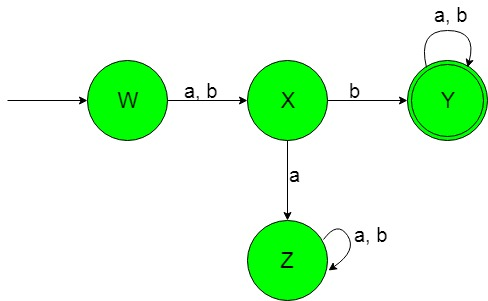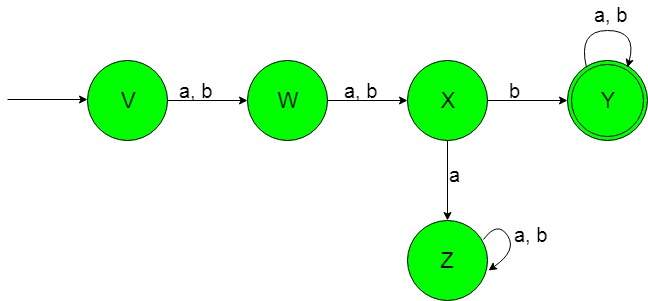TOC | Designing Deterministic Finite Automata (Set 8)

Prerequisite: Designing finite automata
In this article, we will see some designing of Deterministic Finite Automata (DFA).

Problem-1: Construction of a minimal DFA accepting a set of strings over {a, b} in which the second symbol from left-hand side is always ‘b’.
Explanation: The desired language will be like:

L1 = {ab, aba, abaa, bb, bb, bbbb, ...........}

Here as we can see that each string of the language containing ‘b’ as the second symbol from left-hand side but the below language is not accepted by this DFA because some of the string of the below language does not contain ‘b’ as the second symbol from the left-hand side.

L2 = {ba, ba, babaaa..............}

This language L2 is not accepted by this required DFA because it does not contain ‘b’ as the second symbol from the left-hand side.
The state transition diagram of the desired language will be like below:In the above DFA, The state ‘W’ is the initial state which on getting either ‘a’ or ‘b’ as the input it transit to a state ‘X’. The state ‘X’ on getting ‘b’ as the input it transits to the final state ‘Y’ and on getting ‘a’ as the input it transit to a dead state ‘Z’.

The final state ‘Y’ on getting either ‘a’ or ‘b’ as the input it remains in the state of itself. The dead state ‘Z’ is called dead because it can not go to the final state on getting any of the input alphabets.

Note: The number of states in the above DFA is (n+2), where ‘n’ is the number from the left-hand side of the string used in the above language.

Problem-2: Construction of a minimal DFA accepting a set of strings over {a, b} in which the third symbol from left-hand side is always ‘b’.
Explanation: The desired language will be like:

L1 = {aab, baba, aabaa, bbb, abb, bbbb, ...........}

Here as we can see that each string of the language containing ‘b’ as the third symbol from left-hand side but the below language is not accepted by this DFA because some of the string of the below language does not contain ‘b’ as the third symbol from the left-hand side.

L2 = {baa, aba, baabaaa..............}

This language L2 is not accepted by this required DFA because it does not contain ‘b’ as the third symbol from the left-hand side.
The state transition diagram of the desired language will be like below:In the above DFA, The state ‘V’ is the initial state which on getting either ‘a’ or ‘b’ as the input it transits to the state ‘W’. The state ‘W’ is a state which on getting either ‘a’ or ‘b’ as the input it transits to a state ‘X’. The state ‘X’ on getting ‘b’ as the input it transits to the final state ‘Y’ and on getting ‘a’ as the input it transit to a dead state ‘Z’.

The final state ‘Y’ on getting either ‘a’ or ‘b’ as the input it remains in the state of itself. The dead state ‘Z’ is called dead because it can not go to the final state on getting any of the input alphabets.

Note: The number of states in the above DFA is (n+2), where ‘n’ is the number from the left-hand side of the string used in the above language.My Personal Notes arrow_drop_upCheck out this Author's contributed articles.

If you like GeeksforGeeks and would like to contribute, you can also write an article using contribute.geeksforgeeks.org or mail your article to contribute@geeksforgeeks.org. See your article appearing on the GeeksforGeeks main page and help other Geeks.

Please Improve this article if you find anything incorrect by clicking on the "Improve Article" button below.

Article Tags :

Be the First to upvote.

Please write to us at contribute@geeksforgeeks.org to report any issue with the above content.